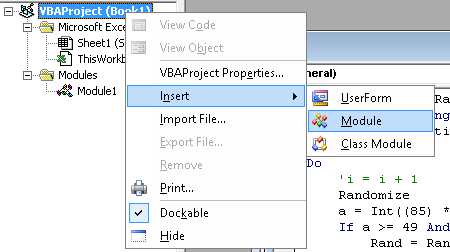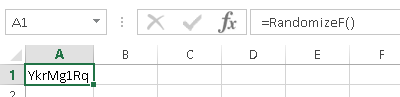### Random number

For any reason, if you need to generate a alphanumeric password, the VBA code below will be able to help you.

1. Press Alt+F11 to bring up the Visual Basic Editor.
2. Right click the book object and select Insert > Module.3. Paste the code below into the Module window.
4. At any cell enter function =RandomizeF()5. This code will skip Capital "I", Capital "O", small letter "l", small letter "o" because I use this code to generate random password. In order to avoid confuse, I decide to remove these letter.

*

Public Function RandomizeF()

Dim Rand As String
Application.Volatile

Do
'i = i + 1
Randomize
a = Int((85) * Rnd + 38)
If a >= 49 And a <= 57 Then
Rand = Rand & Chr(a)
ElseIf a >= 65 And a <= 72 Then
Rand = Rand & Chr(a)
ElseIf a >= 74 And a <= 78 Then
Rand = Rand & Chr(a)
ElseIf a >= 80 And a <= 90 Then
Rand = Rand & Chr(a)
ElseIf a >= 97 And a <= 107 Then
Rand = Rand & Chr(a)
ElseIf a >= 109 And a <= 110 Then
Rand = Rand & Chr(a)
ElseIf a >= 112 And a <= 122 Then
Rand = Rand & Chr(a)
End If
Loop Until Len(Rand) = 8
RandomizeF = Rand
End Function

---